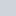# Get the Current Timestamp in JavaScript

Author — Nitish Kumar

As a developer, every now and then, you will need to get the current timestamp in JavaScript. It allows us to represent both the current date and time as a single number. There are many ways to get the current timestamp or epoch time or number of seconds since January 1, 1970 00:00:00 UTC.

In this tutorial, we will discuss all these methods along with their pros and cons in detail.

## Using Date.now() to Get the Current Timestamp

The Date.now() method returns the number of milliseconds that have elapsed since January 1, 1970 00:00:00 UTC. You can divide the returned value with 1000 to get the number of seconds that have passed during this time.

Here is an example which uses `Date.now()` to get the current timestamp:

JavaScript

``````var timestamp = Math.floor(Date.now() / 1000);

// Output 1516131855 (when I ran it)
console.log(timestamp);``````

It is important to remember that we need to use `Math.floor()` instead of `Math.round()` to convert the result after division into an integer. This is because timestamp is defined as number of second that have passed since January 1, 1970 00:00:00 UTC. Using `Math.round()` will give incorrect results because this method will always round the resulting value to the closest integer which can increase the value of reported timestamp by 1. Using `Math.floor()` will give us the correct result after truncating any fractional seconds that might have passed.

This method is not supported by IE8 and below. Other than that, using it is perfectly fine.

## Using Date.getTime() to Get the Current Timestamp

The `Date.getTime()` method returns the numeric value corresponding to the number of milliseconds that have passed since the unix epoch up to the point of time specified by the calling Date object.

JavaScript

``````var timestamp = Math.floor(new Date().getTime() / 1000);

// Output 1516163648 (when I ran it)
console.log(timestamp);``````

The value returned by this method is independant of the timezone. This means that it will return same value for clients in different timezones.

One advantage of using `Date.getTime()` over `Date.now()` is that it also has browser support for Internet explorer. On the other hand, `Date.getTime()` results in creation of an unnecessary Date object.

## Using Date.valueOf() to Get the Current Timestamp

The `Date.valueOf()` method also returns the number of milliseconds that have passed since the unix epoch up to the point of time specified by the calling Date object.

JavaScript

``````var timestamp = Math.floor(new Date().valueOf() / 1000);

// Output 1516164445 (when I ran it)
console.log(timestamp);``````

This method is functionally equivalent to the `Date.getTime()` method. It is generally called internally by JavaScript and not explicitly by developers. For example, the unary plus operator in the following example automatically triggers the `valueOf()` method and returns the timestamp.

JavaScript

``````var timestamp = Math.floor( + new Date() / 1000);

// Output 1516164709 (when I ran it)
console.log(timestamp);``````

Just like the `Date.getTime()` method, the `Date.valueOf()` method is also supported by all browsers including IE8 and below.

## Quick Summary

Let’s recap everything that we have covered in this tutorial.

1. You can get the current timestamp in JavaScript by using the `Date.now()` method. It gives you the timestamp without creating unnecessary Date objects. However, it is not supported by IE8 and below.
2. If you want to support old browsers, you should use `Date.getTime()` to get the current timestamp. One disadvantage of this method is that it will create an extra Date object.
3. You can also use the `Date.valueOf()` method to get the current timestamp in JavaScript. It is functionally equivalent to the `Date.getTime()`. However, it is generally called internally by JavaScript when it needs to convert a date to a number.

Let me know if there is anything that you would like me to clarify in this tutorial. Also, you are more than welcome to comment if you know other techniques to get the current timestamp in JavaScript.

Rate this post —(No Ratings Yet)Loading...
Subscribe
Notify of